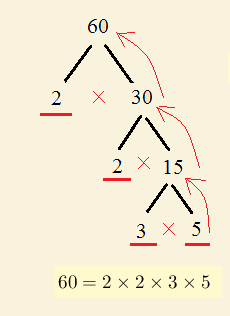# Prime Factorization in Maths - Grade 7 Maths Questions With Detailed Solutions

What is prime factorization of numbers in maths? Grade 7 maths questions are presented along with detailed solutions. Detailed Solutions and explanations are included.

What is prime factorization of numbers in maths?

What are factors?

Factors are numbers that when multiplied together gives another number.
Examples:
1) 2 and 5 are factors of 10 because 2 � 5 = 10.
2) 2, 3, 4 and 6 and factors of 12 because
2 � 2 � 3 = 12
4 � 3 = 12
2 � 6 = 12
What is prime factorization?

Prime factorization is to factor a number into prime numbers ONLY.
Examples:
10 = 2 � 5 , both factors 2 and 5 are prime numbers
12 = 2 � 2 � 3 , both factors 2 and 3 are prime numbers
42 = 2 � 3 � 7 , all factors 2, 3 and 7 are prime numbers

How to find prime factorization of any whole number?

Prime factorization is unique and can be achieved by successive division using the prime numbers: 2, 3, 5, 7, 11, ...

Examples:
1 - Find the prime factorization of 30.
STEP 1:
Divide the given number by the first prime number 2 if divisible (if not by the next prime numbers 3, 5, 7 ... and so on):

30 � 2 = 15

STEP 2:
Divide the result of the division in step 1 by the first prime number 2 if possible (if not by the next prime numbers 3, 5, 7 ... and so on):
15 � 3 = 5

STEP 3: The result of the division in step 2 is the prime number 5. We stop.
The prime factorization of 30 involves all divisors and the last result: 30 =
2 � 3 � 5

2 - Find the prime factorization of 60.
We present the "tree method" of prime factorization which is based on the method of division but its presentation is slightly different. see below example below..

Answer the following questions on prime factorization.

1. Which of the following is not a prime factorization?
a) 20 = 2�10 , b) 14 = 2�7 , c) 64 = 4 3 , d) 120 = 2 3 � 15
2. What is the prime factorization of the following numbers?
a) 28 , b) 32 , c) 100 , d) 126 , e) 900
3. Two numbers A and B are given by: A = 2 3 � 5 2 � 11 and B = 2 2 � 5 � 13. What is the prime factorization of A�B?
4. Find the prime factorization of 100 and 70 and then the prime factorization of 7000 knowing that 7000 = 100 � 70
5. a) Find the prime factorization of 10.
b) Use the result in part a) and the fact that 100 = 10 � 10 to find the prime factorization of 100.
c) Use the result in part a) and the fact that 1000 = 10 � 10� 10 to find the prime factorization of 1000.
d) Use the results in parts a), b) and c) to find a pattern of prime factorization and find the prime factorization of 1000,000.
6. Detailed Solutions and explanations are included.